# Orchard

One-eighth of the trees in the fruit plant in winter froze and one-twelfth of damaged disease and pests. Healthy trees remained 152. Is it enough to supply 35 trees to restore the original number of trees in the orchard?

n =  192
v =  40

### Step-by-step explanation:

1/8n + 1/12n + 152 = n

1/8•n + 1/12•n + 152 = n

19n = 3648

n = 192

Our simple equation calculator calculates it.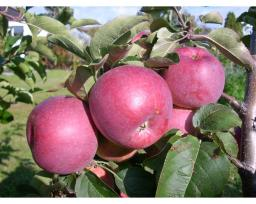Did you find an error or inaccuracy? Feel free to write us. Thank you!Aj
this is a really good cauculatorAj
thank you very muchTips to related online calculators
Need help to calculate sum, simplify or multiply fractions? Try our fraction calculator.
Do you have a linear equation or system of equations and looking for its solution? Or do you have a quadratic equation?

## Related math problems and questions:

• Orchard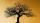3/5 of the trees in the orchard are apples, 1/3 are cherries and the remaining five trees are pear. How many trees are in the orchard?
• Orchard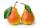Three-fifths of the orchard are apple trees, one-third of the trees are cherries. The remaining 5 trees are pears. How many trees are in the orchard?
• TreesFrom the total number of trees in the orchard, there are two-fifths pearls and apples are three eighty. The rest of the trees are 9 ceremonial. How many trees are in the set?
• Unknown numberFind the unknown number equal to a quarter of a fifth of a number, which is by 152 more than an unknown number.
• Trees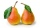Two-fifths of the trees in the orchard are apple trees, one-sixth a pear. The remaining 26 trees are cherries. How many pears are in the orchard?
• Trees - orchardThe 3/5 trees are apple trees and 1/3 of the trees are cherries. The remaining 5 trees are pear trees. How many trees are in the orchard.
• Disease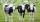Farmers'cooperative had a supply of feed for 50 cows to 6 days. Cows got insidious disease after 4 days, 10 of them died. How many total days that farmers had feed for cows?
• Trees avgThe worker planted 96 trees on Monday, 120 on Tuesday, and 61 trees on Wednesday. How many trees did he plant on Thursday if he averaged 105 trees per day?
• Trees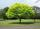Loggers wanted to seed more than 700 and less than 800 trees. If they seed in rows of 37, left them 8 trees. If they seed in rows of 43, left the 11 trees. How many trees must seed ?
• QuotientFind quotient before the bracket - the largest divisor 51 a + 34 b + 68 121y-99z-33
• Plums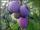In the bag was to total 136 plums. Igor took 3 plums and Mary took 4/7 from the rest. How many plums remained in the bag?
• Trees3/5 trees are apples, cherries are 1/3. 5 trees are pear. How many is the total number of trees?
• There 14There are 250 people in a museum. 2/5 of the 250 people are girls 3/10 of the 250 people are boys The rest of the 250 people are adults Work out the number of adults in the museum.
• Even / odd numbers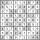a / Using variable n write two consecutive odd numbers. b / the sum of three consecutive odd numbers is 333. What are this numbers?
• An orchard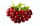In an orchard, there are 28 apple trees 27 orange trees and 14 cherry tree. What is the ratio of apple trees to cherry trees?
• On the farm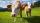On the farm were housed 70 heads of cattle with food supply for 180 days. After 60 days, 10 head of cattle sold, and for the next 30 days they sold 10 more. How many days is sufficient supply of feed for the remaining cattle?
• School year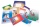At the beginning of the school year, 396 notebooks and 252 textbooks are ready to be distributed in the classroom. All pupils receive the same number of notebooks and the same amount of textbooks. How many pupils are there in the class if you know that th